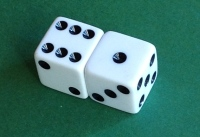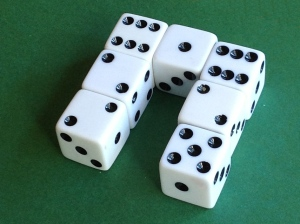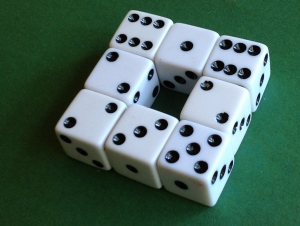# Euler characteristic with dice

For any convex solid, VE + F = 2 where V is the number of vertices, E the number of edges, and F the number of faces. The number 2 in this formula is a topological invariant of a sphere, called its Euler characteristic. But if you compute the Euler characteristic for a figure with a hole in it, you get a different value. For a torus (the surface of a doughnut) we get VE + F = 0.

You can demonstrate this with eight 6-sided dice. A single die has 8 vertices, 12 edges, and 6 faces, and so VE + F = 2. Next join two dice together along one face.Before joining, the two dice separately have 16 vertices, 24 edges, and 12 faces. But when we join them together, we have 4 fewer vertices since 4 pairs of edges are identified together. Similarly, 4 pairs of edges are identified, and 2 faces are identified. So the joined pair now has 12 vertices, 20 edges, and 10 faces, and once again VE + F = 2.

We can keep on adding dice this way, and each time the Euler characteristic doesn’t change. Each new die adds 4 vertices, 8 edges, and 4 faces, so VE + F doesn’t change.But when we join the dice into a circle, the Euler characteristic changes when we put the last die in place.The last die doesn’t change the total number of vertices, since all its vertices are identified with previous vertices. The last die adds 4 edges. It adds a net of 2 faces: it adds 4 new faces, but it removes 2 existing faces. So the net change to the Euler characteristic is 0 – 4 + 2 = -2. The last die lowers the Euler characteristic from 2 to 0.

Exercise: Use a similar procedure to find the Euler characteristic of a two-holed torus.## 4 thoughts on “Euler characteristic with dice”

1. In your second example, when you join the two dice, why don’t we fall back to the one die example? On the picture, I see a rectangular parallelepiped, 6 faces, 8 vertices and 12 edges.

2. Nicholas: I’m retaining the internal vertices and edges in my counting. So the lines you see where the dice join count as edges. And where two corners are joined, you now have one vertex.

3. In the last example(when you finish the circle with 8 dice), instead of doing the calculations with unitary dies, if I try to work with the final shape then the formula doesn’t work:
V – 16
E – 24
F – 10
V-E+F = 2
What am I doing wrong?